[top.htm]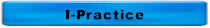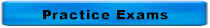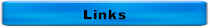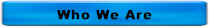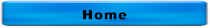Answers to More Wastewater Treatment Questions

 Matching:  A predominance of these microorganisms indicates 1.  Amoeba A.  Fast settling biomass and pin floc 2.  Flagellates B.  Good settling biomass 3.  Ciliates C.  Slow settling biomass and stragglers 4.  Rotifers 5.  Nematodes

1.  Amoeba = Slow settling biomass and stragglers

2.  Flagellates = Slow settling biomass and stragglers

3.  Ciliates = Good settling biomass

4.  Rotifers = Slow settling biomass and stragglers

5.  Nematodes = Slow settling biomass and stragglers

Which of the following are associated with ashing in the final clarifier(s) of an activated sludge plant?

 Variable High Low F : M Low SRT High MCRT High Sludge Age High Foam on AT Dark Protozoa Rotifers

Chlorine ton cylinders at room temperature will deliver gas at a maximum rate of

150 lb cylinders        Ton Containers

A.  10 pounds per day      100 pounds per day

B.  25 pounds per day      250 pounds per day

C.  40 pounds per day      450 pounds per day

D.  150 pounds per day    2000 pounds per day

The correct answer is C

Which of the following lab tests is used to determine the total amount of nitrogen in our effluent?

A.  TN

B.  TKN

C.  NO3

D.  NH3

The correct answer is A

According to USEPA, the standard secondary removal efficiency is

A.  15%

B.  30%

C.  85%

D.  95%

The correct answer is C

A special thanks to Courtney Cswercko, Iowa DNR, for her presentation at the March 6, 2009 AceOps Annual Conference in Council Bluffs, IA!!!  Her presentation is the source of this question.

The maximum holding time for e. Coli samples according to Standard Methods is

A.  30 minutes

B.  60 minutes

C.  6 hours

D.  36 hours

The correct answer is C

A special thanks to Courtney Cswercko, Iowa DNR, for her presentation at the March 6, 2009 AceOps Annual Conference in Council Bluffs, IA!!!  Her presentation is the source of this question.

Which of the following is used for process control for activated sludge?

A.  MCRT

B.  Surface settling rate

C.  Volatile acids to alkalinity ratio

D.  Weir overflow rate

The correct answer is A

B and D are used for process control for clarifiers

C is used for process control for anaerobic digesters

The correct answer is C

The correct answer is D

The correct answer is E

It is not B because the trickling filters are not in service

A.  5

B.  6

C.  4

D.  1

E.  3

Is this how a lagoon should look?

No, the surface should be open

What is the cause of this?

Duckweed

What is the minimum recommended velocity in non-aerated grit chambers?

A.  0 fps

B.  1 fps

C.  2 fps

D.  10 fps

The correct answer is B.  1 fps

C is the minimum recommended velocity in sanitary sewers

D is the maximum recommended velocity in sanitary sewers

A settleometer is used to measure

A.  Rate of settling in a final clarifier

B.  Rate of settling in a primary clarifier

C.  Sludge depth in a final clarifier

D.  Sludge depth in a primary clarifier

The correct answer is A.  Rate of settling in a final clarifier

B is incorrect because a settleometer is used to measure the settling rate in a final clarifier

C is incorrect because a sludge judge, not a settleometer, is used to measure the sludge depth

D is incorrect because a settleometer is not used to measure the sludge depth in a primary clarifier

Which of the following valves only permits flow to move in one direction?

A.  Butterfly

B.  Check

C.  Gate

D.  Plug

The correct answer is B

The specific gravity of a colloidal solid is very close to 1.  This means that the colloid

A.  Will float within an hour

B.  Will settle within an hour

C.  Won't settle or float

The correct answer is C

The specific gravity of settleable solid is

A.  1

B.  Greater than 1

C.  Less than 1

The correct answer is B

The specific gravity of a floatable solid is

A.  1

B.  Greater than 1

C.  Less than 1

The correct answer is C

The specific gravity of chlorine gas is

A.  1

B.  Greater than 1

C.  Less than 1

The correct answer is B

 Matching 1.    Calcium A.  C 2.    Carbon B.  S 3.    Chlorine C.  P 4.    Copper D.  K 5.    Fluorine E.  O 6.    Iron F.   Na 7.    Hydrogen G.  N 8.    Lead H.  Mg 9.    Magnesium I.    Ca 10.  Manganese J.   Cl 11.  Nitrogen K.  Cu 12.  Potassium L.   Mn 13.  Sodium M.   Fe 14.  Oxygen N.   H 15.  Phosphorus O.   Pb 16.  Sulfur P.   Fl

1.  I

2.  A

3.  J

4.  K

5.  P

6.  M

7.  N

8.  O

9.  H

10.  L

11.  G

12.  D

13.  F

14.  E

15.  C

16.  B

Which of the following is correct?

A.  A is the follower and B is the lantern ring

B.  A is the lantern ring and B is a packing ring

C.  A is a packing ring and B is the lantern ring

D.  A is a packing ring and B is the follower

The correct answer is C.  A is a packing ring and B is the lantern ring

pH measures

A.  H+

B.  OH-

C.  O2

D.  Phosphorus

The correct answer is A.  H+  -- pH measures the hydrogen ion concentration

How do you know that your pump is cavitating?

A.  It doesn't sound right

B.  It runs hot

C.  It won't start

D.   All of the above

The correct answer is A.  It doesn't sound right.

A pump that's cavitating sounds like it's pumps rocks or gravel.

A chlorine atom is composed of

A.  Electrons

B.  Neutrons

C.  Protons

D.  All of the above

The correct answer is D.  All of the Above

Throttling the discharge valve on a centrifugal pump will the likely ______ temperature of the motor and ______ the temperature of the pump

A.  Increase, increase

B.  Increase, reduce

C.  Reduce, increase

D.  Reduce, reduce

The correct answer is C.  Reduce, increase

A, B, and D are incorrect because throttling the discharge valve on a centrifugal pump will the likely reduce temperature of the motor and increase the temperature of the pump

The chemical symbol for chlorine gas is

A.  Cl

B.  Cl-

C.  Cl2

D.  HOCl

The correct answer is C.  Cl2

A is incorrect because this is chemical symbol for chlorine not chlorine gas

B is incorrect because this is the chemical symbol for chloride

D is incorrect because this is the chemical formula for hypochlorite

Which of the following is the indicator used for pathogens?

A.  Amoebic dysentery

B.  E. coli

C.  Salmonella typhosa

D.  V. comma

The correct answer is B.  E. coli

A is incorrect because this is a pathogen, not an indicator

C is incorrect because this is a pathogen (cholera), not an indicator

D is incorrect because this is a pathogen (typhoid), not an indicator

Pump suction and discharge pressures are usually measured in

A.  gpm

B.  kWh

C.  mg/L

D.  psi

The correct answer is D.  psi

A is incorrect because this is a measure of flow

B is incorrect because this is a measure of energy used

C is incorrect because this is a measure of concentration

Which of the following is the correct formula for chlorine in water?

A.  Cl2 + H2O → HOCl + HCl

B.  NaOCl + H2O → HOCl + NaOH

C.  Ca(OCl)2 + 2H2O → 2HOCl + Ca(OH)2

D.  HOCl → H+ + OCl-

E.  All of the above

The correct answer is E.  All of the Above

Which of the following is the correct wavelength of UV for disinfection?

A.  253.7 nm

B.  372.5 nm

C.  537.2 nm

D.  725.3 nm

The correct answer is A

Which of the following describes flocculation?

A.  Suspended solids that are too small to settle

B.  Quiescent conditions that allow settleable solids to settle

C.  Neutralizing charges

D.  Growth of smaller solids into larger masses that will settle

The correct answer is D.  Growth of smaller solids into larger masses that will settle

A is incorrect because this describes colloids

B is incorrect because this describes sedimentation

C is incorrect because this describes coagulation

Hypoxic zones are caused by high levels of

A.  Colloidal suspended solids

B.  Dissolved O2

C.  Nitrogen & phosphorus

D.  Pathogens

The correct answer is C.  Nitrogen & phosphorus

A is incorrect because high levels of colloidal suspended solids cause turbidity problems

B is incorrect because hypoxic zones have low or no dissolved oxygen

D is incorrect because a breakdown in the disinfection process would lead to high levels of pathogens

The PE for flow is ____ gpcd and PE for BOD is _____ lbs BOD/person/day.

A.  0.17 for both

B.  0.17 and 100

C.  100 and 0.17

D.  100 for both

The correct answer is C 100 and 0.17

B, C, D are incorrect because the PE (population equivalent) for flow is 100 gpcd (gallons per capita per day and 0.17 lbs BOD/person/day

1.  D

2.  A

What is the minimum recommended velocity in sanitary sewers?

A.  0 fps

B.  1 fps

C.  2 fps

D.  10 fps

The correct answer is C.  2 fps

C is the minimum recommended velocity in non-aerated grit chambers

D is the maximum recommended velocity in sanitary sewers

For questions or comments, call 515-313-1159

Home